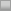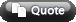View Single Post03-05-2008, 02:44 AM #6 stealthcow adept   Join Date: Jul 2004 Location: i <3 Toronto Posts: 1,095 Re: Maximizing EV to Find a GTO Betsize Hey guys, A few questions. First I ordered the mathematics of poker but it hasn't arrived yet. Can someone explain to me the "indifference equation and how it goes to 1/(1+S) ? Shouldn't it be 1/(1+2s), where 1 = the pot size? 2nd, I tried to break both the c/cer (player 1) and the bettor (player 2) into their playing ranges. We assume that at this point of time player 2 has already bet. P = current pot size on the river S = Bet size For the check caller: A - range where he beats everything C - He beats our bluffs, loses to our legit hands E - Loses to our bluffs/ everything For the range of the Bettor B - Loses to A, beats C, beats E D - Loses to C, beats E So of course, this notation is such that A > B > C > D > E Also note B + D = 1. So the EV of the guy calling is... B(A(P+S) - CS - ES) + D(A(P+S) + C(P+S) - E(S)) now sub in D = 1-b and begin to simplify B(AP + AS - CS - ES) + (1-B)(AP + AS + CP + CS - ES) BAP + BAS - BCS - BES + (AP + AS + CP + CS - ES) - (BAP + BAS + BCP +BCS - BES) BAP + BAS - BCS - BES + AP + AS + CP + CS - ES - BAP - BAS - BCP - BCS + BES we can eliminate some terms AP + AS + CP + CS - ES - BCP - 2BCS now combine terms with P and those with S in it P(A+C-BC) + S(A+C-E-2BC) I think this is all sound, although i'm a bit confused on what A+C+E should add up to. If its supposed to add up to less than 1, then I think this is okay, if it should add up to 1, then i'm confused. Because from here i'd say that when we bet our ev is (% vill folds)*pot size - (1- % vill folds)*(Caller's EV) If villain folding is just (1-A-C-E), then to solve this we just need to say that P(1-A-C-E) - (A+C+E)(P(A+C+BC) + S(A+C-E-2BC)) = 0. From here you can get the value of S/P, given you know A,B,C and E i'm pretty 50/50 on if i made a mistake somewhere in here. Please let me know if i did, as i'd like to learn how to do these calculations and improve on them. thanks, stealthcow-